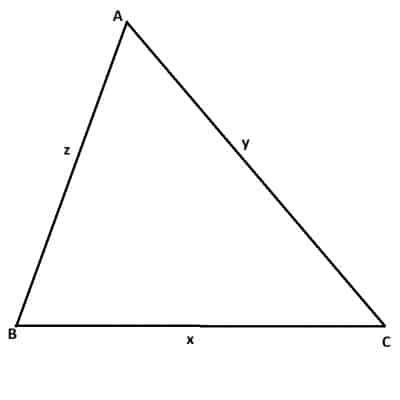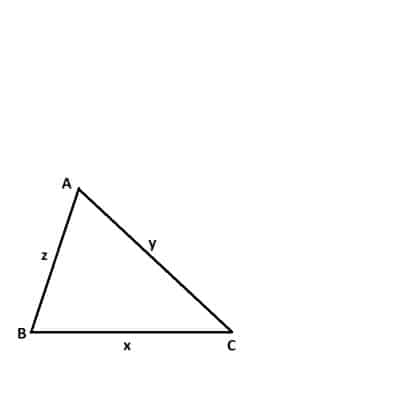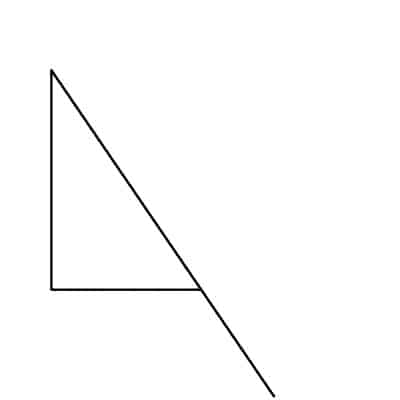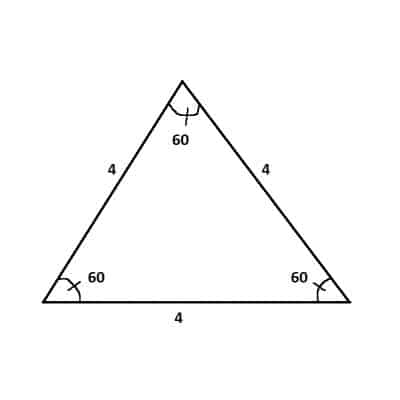# What Is The Triangle Inequality? (3 Key Concepts To Know)

When working with triangles, we often want to calculate and compare side lengths (in addition to angles).  The triangle inequality is one tool that we can use to help us.

So, what is the triangle inequality?  The Triangle Inequality relates the lengths of the three sides of a triangle. Specifically, the Triangle Inequality states that the sum of any two side lengths is greater than or equal to the third side length.  If the side lengths are x, y, and z, then x + y >= z, x + z >= y, and y + z >= x.

Of course, equality only happens with a “degenerate” triangle, which is one with zero area (and where the longest side overlaps with the two shorter sides).

Let’s get started.

## What Is The Triangle Inequality?

The Triangle Inequality Theorem states that:

“For a triangle with side lengths x, y, and z, the sum of any two side lengths will be greater than or equal to the third side length.”

(A more general statement about distances is that for real numbers x and y, |x + y| <= |x| + |y|).

In symbols, this means that all three of the following equations hold true:

• x + y >= z
• x + z >= y
• y + z >= x

Note that this has a specific implication that relates the perimeter of a triangle to its side lengths.

### Triangle Inequality & Perimeter Of A Triangle

For a triangle with side lengths x, y, and z, the perimeter is simply x + y + z.  We can use the Triangle Inequality to show:

• x + y + z  [perimeter of triangle]
• =(x + y) + z  [group the x and y terms together]
• >= z + z  [x + y >= z, by the Triangle Inequality]
• = 2z

We can prove something similar for x and y.  That is, x + y + z >= 2x and x + y + z >= 2y.

Essentially, this tells us that the perimeter of a triangle is at least twice the longest side.

### Why Is The Triangle Inequality Theorem True? (Proof Of Triangle Inequality)

Let’s look at two different ways to think about the Triangle Inequality:

• An intuitive “proof” using the concept of distance between two points.
• A rigorous proof using algebra.

#### Intuitive “Proof” Of The Triangle Inequality

Consider any triangle, such as the one pictured below.For a triangle with side lengths x, y, and z, the Triangle Inequality tells us that x + y >= z (the sum of any two side lengths is greater than or equal to the length of the third side).

Imagine that the area inside and outside of the triangle is filled with deep water (and you don’t know how to swim!)  To get from point A to point C, you will have to walk around the perimeter of the triangle.

There are two ways you can do it.  You can either:

• walk from A to B (a distance of z) and then from B to C (a distance of x), for a total distance of x + z.
• walk from A to C (a distance of y)

The shortest distance between two points is a straight line, so y is the shortest distance between A and C.  This means that x + z must be a longer distance than y, so x + z >= y (the Triangle Inequality).

Now that you understand the intuition behind the theorem, here is how to prove the Triangle Inequality Theorem with a rigorous argument.

#### Rigorous Proof Of The Triangle Inequality

Consider the triangle below, with its three vertices labeled A, B, C and its three side lengths labeled x, y, z (x is opposite vertex A, y is opposite vertex B, and z is opposite vertex C).Here we have a labeled triangle with vertices A, B, and C with side lengths x, y, and z.

First, draw a dashed line that extends side BA of the triangle, out to a distance of y, to the point D (as shown below).

Next, draw a dashed line connecting point D to point C (as shown below).

Then, note that the sides AD and AC in the top-right triangle are congruent, since they have the same length y.  This means that their opposite angles (angle ACD and angle D) must be congruent (call this angle measure “t”).

Now, note that angle BCD in the large triangle is greater than the angle ACD in the top-right triangle.  Also, recall from earlier that angle ACD is equal to angle D.  This means that:

• angle BCD > angle ACD = angle D

So angle BCD > angle D.

Finally, note that in the large triangle, the opposite side of angle BCD is y + z, and the opposite side of angle D is x.

Since angle BCD > angle D, then y + z > x (opposite side must be larger for a larger angle).

We can prove the other statements (x + y > z and x + z > y) with similar arguments.

### Why Is The Triangle Inequality Theorem Important?

The Triangle Inequality theorem is important because we can use it to figure out whether three side lengths can form a viable triangle or not.There are some combinations of three side lengths that do not form any valid triangle, no matter how you rearrange them.

The Triangle Inequality is also useful for establishing bounds for sums when working with absolute values or other measures.

### Does The Triangle Inequality Work With Subtraction?

We can restate the triangle inequality theorem with subtraction if necessary.  For example, we can get two subtraction inequality statements from:

• x + y >= z

For the first one, subtract y from both sides to get:

• x >= z – y

For the second one, subtract x from both sides to get:

• y >= z – x

We can also use the other two Triangle Inequality statements (x + z >= y and y + z >= x) to come up with four other statements:

• x >= y – z
• z >= y – x
• y >= x – z
• z >= x – y

### When Does Equality Hold In The Triangle Inequality?

Equality holds in the Triangle Inequality in the special case of a degenerate triangle.  All this means is that the shorter sides (let’s call them x and y here) overlap with the longest side z.

This triangle is degenerate because it has an area of zero (no height!) and all of the angles are 0, 0, and 180 degrees.

In all other cases, the Triangle Inequality is strict; that is, x + y > z.

### Examples Of The Triangle Inequality

Let’s look at some examples of how we can use the Triangle Inequality to decide if a combination of three side lengths is possible or not.

#### Example 1: An Impossible Triangle

Consider the following side lengths:

• x = 2
• y = 3
• z = 6

Can we construct a triangle with these side lengths?

The answer is no.  The Triangle Inequality shows us why:

• x + y >= z  [by the Triangle Inequality]
• 2 + 3 >= 6 (?)
• 5 >= 6 (?)

This final statement is not true, so it contradicts the Triangle Inequality.  There is no triangle with side lengths 2, 3, and 6.

*Note: it is true that 2 + 6 > 3 and 3 + 6 > 2, but this is not enough!  All three statements from the Triangle Inequality must hold, or else the triangle is impossible with a given set of three side lengths!

#### Example 2: Another Impossible Triangle

Consider the following side lengths:

• x = 4
• y = 10
• z = 5

Can we construct a triangle with these side lengths?

The answer is no.  The Triangle Inequality shows us why:

• x + z >= y  [by the Triangle Inequality]
• 4 + 5 >= 10 (?)
• 9 >= 10 (?)

This final statement is not true, so it contradicts the Triangle Inequality.  There is no triangle with side lengths 4, 5, and 10.

#### Example 3: A Triangle That Can Exist

Consider the following side lengths:

• x = 2
• y = 3
• z = 4

Can we construct a triangle with these side lengths?

The answer is yes.  All 3 statements of the Triangle Inequality are satisfied:

• 2 + 3 >= 4 (this is true)
• 2 + 4 > = 3 (this is true)
• 3 + 4 >= 2 (this is true)

#### Example 4: Another Triangle That Can Exist

Consider the following side lengths:

• x = 4
• y = 4
• z = 4

Can we construct a triangle with these side lengths?

The answer is yes.  All 3 statements of the Triangle Inequality are satisfied, since:

• 4 + 4 >= 4 (this is true)

This specific case is an example of an equilateral triangle, which has three equal side lengths (and thus three equal angle measures, each of 60 degrees).An equilateral triangle with side lengths of 4 (and three angles that are 60 degrees each) is valid and we can construct it.

#### Example 5: A Bounded Interval For The Side Length Of A Triangle

Consider a triangle with sides x, y, and z.  Side lengths y and z are both 1, while x is unknown.

The Triangle Inequality statements tell us:

• x + y >= z
• x + z >= y
• y + z >= x

Or, substituting y = 1 and z = 1:

• x + 1 >= 1
• x + 1 >= 1
• 1 + 1 >= x

Simplifying these inequalities, we get:

• x >= 0
• x >= 0
• 2 >= x

Combining these statements, we get 0 <= x <= 2.

The case x = 0 is the degenerate triangle with side lengths 0, 1, 1.

The case x = 2 is the degenerate triangle with side lengths 2, 1, 1.

The cases 0 < x < 2 give us infinitely many valid triangles with side lengths x, 1, 1.  Note that these are all isosceles triangles (and in the case of x = 1, the triangle is equilateral).

## Conclusion

Now you know what the triangle inequality is and how to use it.  You also know how to prove the statement of the theorem.

You can learn about the uses of inequalities in everyday life here.

You can learn about uses of triangles in real life here.